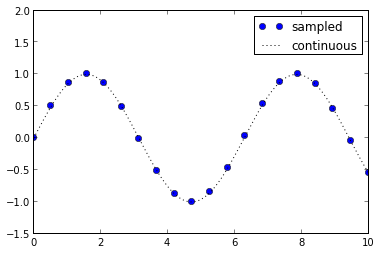# 1. Basic Plotting with Pylab¶

Use the “v2” files in older versions of IPython, e.g. 0.12

# Matplotlib Tutorial: 1. Basic Plot Interface

In this notebook, we will explore the basic plot interface using pylab.plot and pylab.scatter. We will also discuss the difference between the pylab interface, which offers plotting with the feel of Matlab. In the following sections, we will introduce the object-oriented interface, which offers more flexibility and will be used throughout the remainter of the tutorial.

## Setting up IPython

IPython has a built-in mode to work cleanly with matplotlib figures. There are a few ways to invoke it:

1. On startup, you can add a command line argument:

ipython [notebook] --pylab


or:

    ipython notebook --pylab inline


The first can be used with the notebook or with the normal IPython interpreter. The second specifies that figures should be shown inline, directly in the notebook. This is not available with the standard IPython interpreter.

1. After starting IPython, the same can be accomplished through the %pylab magic command:

%pylab


or:

    %pylab inline


The first works in either the interpreter or the notebook; the second should be used in the notebook. This is useful if an IPython session has already started.

We'll take the second route here, and tell IPython we want figures plotted inline:

In :
%pylab inline

Welcome to pylab, a matplotlib-based Python environment [backend: module://IPython.zmq.pylab.backend_inline].


## A first plot: the Pylab interface

Now we're ready for a plot. The %pylab mode we entered above does a few things, among which is the import of pylab into the current namespace. For clarity, we'll do this directly here. We'll also import numpy in order to easily manipulate the arrays we'll plot:

In :
import pylab
import numpy as np


Let's make some simple data to plot: a sinusoid

In :
x = np.linspace(0, 20, 1000)  # 100 evenly-spaced values from 0 to 50
y = np.sin(x)

pylab.plot(x, y)

Out :
[<matplotlib.lines.Line2D at 0x2f723d0>]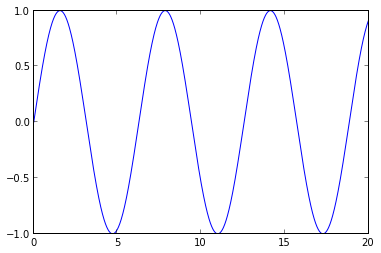### Customizing the plot: Axes Limits

Let's play around with this a bit: first we can change the axis limits using xlim() and ylim()

In :
pylab.plot(x, y)
pylab.xlim(5, 15)
pylab.ylim(-1.2, 1.2)

Out :
(-1.2, 1.2)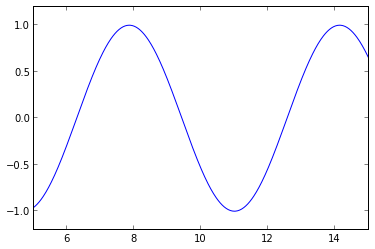### Customizing the plot: Axes Labels and Titles

We can label the axes and add a title:

In :
pylab.plot(x, y)

pylab.xlabel('this is x!')
pylab.ylabel('this is y!')
pylab.title('My First Plot')

Out :
<matplotlib.text.Text at 0x3261390>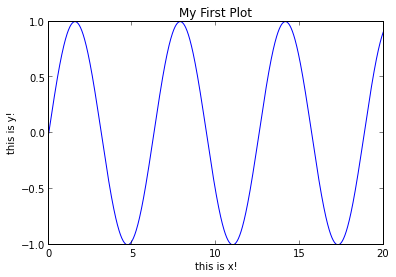Labels can also be rendered using LaTeX symbols:

In :
y = np.sin(2 * np.pi * x)
pylab.plot(x, y)
pylab.title(r'$\sin(2 \pi x)$')  # the r before the string indicates a "raw string"

Out :
<matplotlib.text.Text at 0x2f7d310>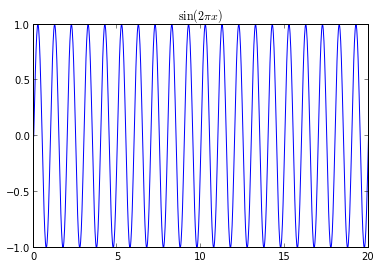### Customizing the plot: Line Styles

We can vary the line color or the line symbol:

In :
pylab.plot(x, y, '-r')  # solid red line ('r' comes from RGB color scheme)
pylab.xlim(0, 10)
pylab.ylim(-1.2, 1.2)

pylab.xlabel('this is x!')
pylab.ylabel('this is y!')
pylab.title('My First Plot')

Out :
<matplotlib.text.Text at 0x38957d0>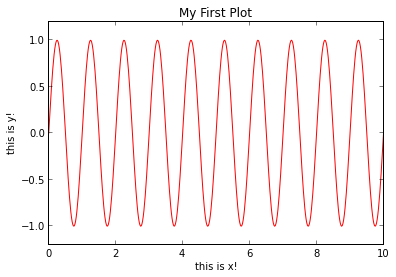Other options for the color characters are:

 'r' = red
'g' = green
'b' = blue
'c' = cyan
'm' = magenta
'y' = yellow
'k' = black
'w' = white


Options for line styles are

 '-' = solid
'--' = dashed
':' = dotted
'-.' = dot-dashed
'.' = points
'o' = filled circles
'^' = filled triangles


and many, many more.

For more information, view the documentation of the plot function. In IPython, this can be accomplished using the ? functionality:

In :
pylab.plot?


Also see the online version of this help: http://matplotlib.org/api/pyplot_api.html#matplotlib.pyplot.plot

### Cusomizing the Plot: Legends

Multiple lines can be shown on the same plot. In this case, you can use a legend to label the two lines:

In :
x = np.linspace(0, 20, 1000)
y1 = np.sin(x)
y2 = np.cos(x)

pylab.plot(x, y1, '-b', label='sine')
pylab.plot(x, y2, '-r', label='cosine')
pylab.legend(loc='upper right')
pylab.ylim(-1.5, 2.0)

Out :
(-1.5, 2.0)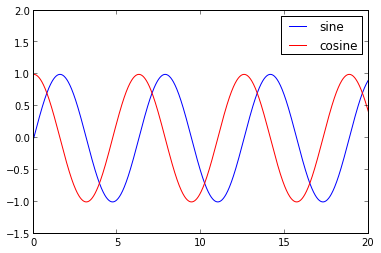### Exercise: Linestyles & Plot Customization

Below are two sets of arrays x1, y1, and x2, y2. Create a plot where x1 and y1 are represented by blue circles, and x2 and y2 are represented by a dotted black line. Label the symbols "sampled" and "continuous", and add a legend. Adjust the y limits to suit your taste.

In :
x1 = np.linspace(0, 10, 20)
y1 = np.sin(x1)

x2 = np.linspace(0, 10, 1000)
y2 = np.sin(x2)

In :
pylab.plot(x1, y1, 'bo', label='sampled')
pylab.plot(x2, y2, ':k', label='continuous')
pylab.legend()

pylab.ylim(-1.5, 2.0)

Out :
(-1.5, 2.0)